## DALL·E $$2$$ - Overview and Applications

MathJax example

In this short blog post, we will discuss the recent DALL·E $$2$$ model.

Discloser: All images in this blog are AI-generated by DALL·E 2.

## Introduction#

DALL·E $$2$$ is a generative model that takes sentences and creates corresponding original images. The model can generate realistic images and art from just text descriptions. It can combine different concepts, attributes, styles, and ideas. At 3.5B parameters, a smaller size than its predecessor DALL·E with (12B), the model is able to generate a better realistic images than the original DALL·E and it’s preferred by human evaluation in both photorealism and caption matching.

In a nutshell, the model takes only an input short text (prompt) and generates a new image by combining the semantic information from the text, such as a related object, background, etc. Figure 1 and Figure 2 below show some creative styles of arts, such as Bauhaus, digital, and pixel created by the model via prompting.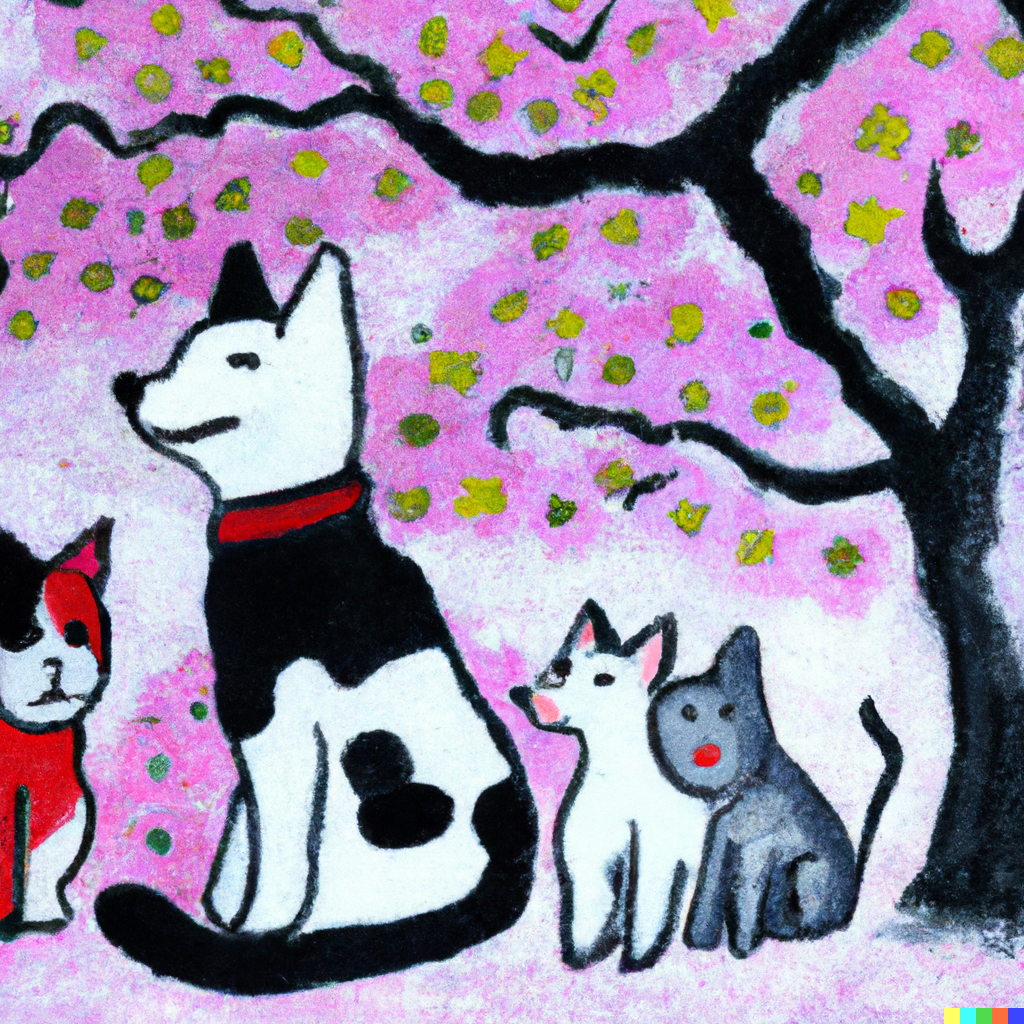Figure 1. (Left) prompt: a picasso style painting of a cat and dog family are enjoying cherry blossom hanami in Japan, (Middle) prompt: cat and dog family are enjoying cherry blossom hanami in Japan, digital art (Right) prompt: cat and dog family are enjoying cherry blossom hanami in Japan, pixel art.Figure 2. (Left) prompt: a Bauhaus style painting of Marilyn Monroe, (Middle) prompt: digital art of Marilyn Monroe and (Right) prompt: pixel art of Marilyn Monroe.

In this short blog, we will take a look at DALL-E 2 and how it manages to create such images as those above. At the highest level, DALL-E 2's works very simply. First, an input text prompt is given to the text encoder that maps the input to a representation space. Next, the prior map the text encoding to the corresponding image using semantic information from the text. Finally, the image encoder generates an image from the text encoding.

## Method#

The generative model DALL·E $$2$$ relies on an inverse CLIP (unCLIP) as the main component to encode image embedding with a prior to generate an image from a given text caption. They rely on CLIP because the model is trained on 400M image-text pairs in an unsupervised manner, and thus the image is embedded with the related text in the same latent space. Figure 1 shows a high-level overview of unCLIP. Note that the dotted box CLIP model is frozen during training.

The model consists of three blocks as shown in the Figure 1 below. The (1) Frozen CLIP, (2) Decoder, and (3) the prior. Next, we will discuss each block in more detail.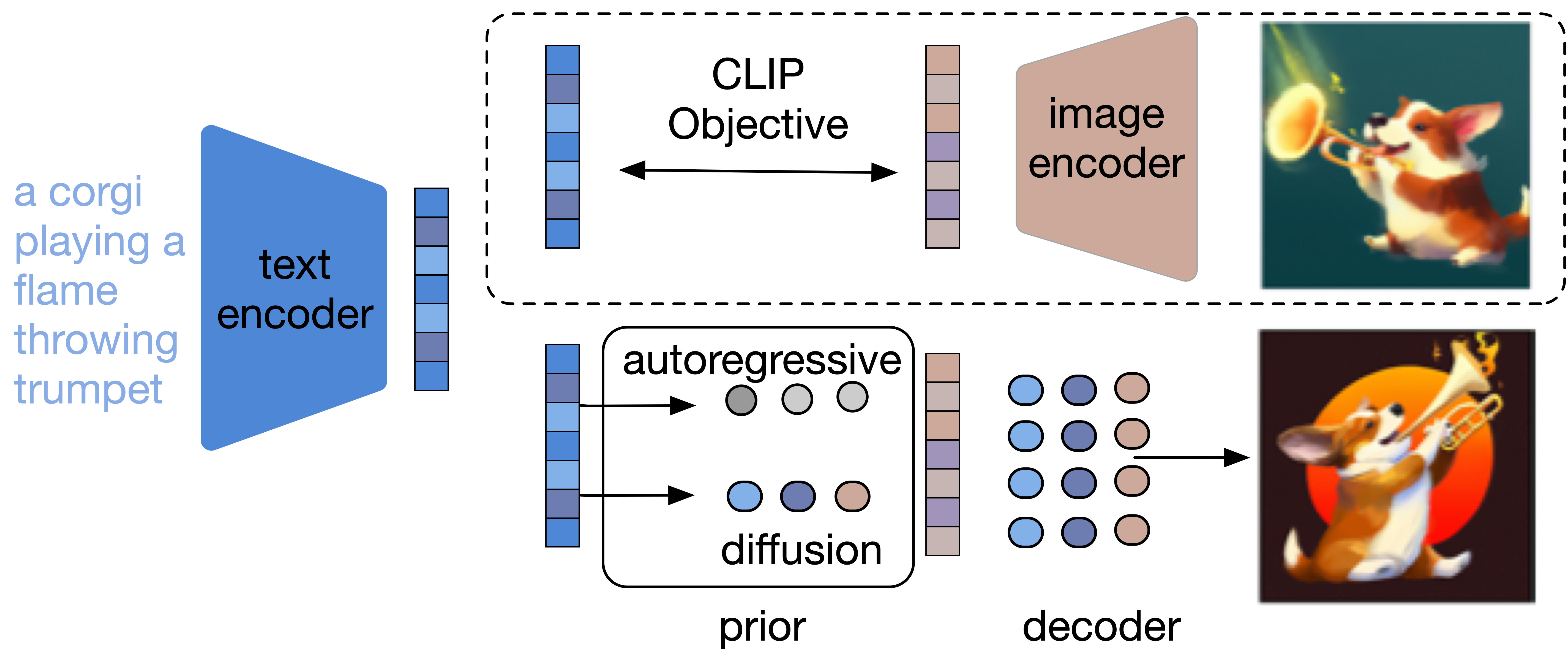Figure 3. A high-level overview of DALL·E $$2$$ (unCLIP). CLIP text embedding is fed to autoregressive or diffusion prior to produce an image embedding, which is used to produce an image embedding that generates a final image. The dotted line indicates CLIP model is frozen during training of the prior and decoder. Figure reproduced from .

CLIP. (Contrastive Language-Image Pre-training) This is a pre-trained model with contrastive loss where the pair of image-text needs to be distinguished from randomly selected sample pairs. CLIP uses available resources across the Internet without human annotation of 400M pairs. CLIP achieves state-of-the-art performance in a wide range of image classification task in zero-short learning.

The CLIP model contains two components a text encoder $$g$$ and image encoder $$f$$. The model during training will use batches of the images and caption pairs as a sample from a large dataset from the web. The model optimizes a contrastive cross-entropy loss, which encourages the high dot product of $$f$$ and $$g$$ if the image and $$c$$ come from the same image captioning pairs, meanwhile, it encourages the low dot product if $$x$$ and $$c$$ come from different image caption pairs. The contrastive cross-entropy loss can be written as:

$\small -\log \frac{\exp \left(f\left(\mathbf{x}_{i}\right) \cdot g\left(\mathbf{c}_{j}\right) / \tau\right)}{\sum_{k} \exp \left(f\left(\mathbf{x}_{i}\right) \cdot g\left(\mathbf{c}_{k}\right) / \tau\right)}-\log \frac{\exp \left(f\left(\mathbf{x}_{i}\right) \cdot g\left(\mathbf{c}_{j}\right) / \tau\right)}{\sum_{k} \exp \left(f\left(\mathbf{x}_{k}\right) \cdot g\left(\mathbf{c}_{j}\right) / \tau\right)}$

Given the optimal value of $$f(x) \cdot g(c)$$, CLIP can be used to generate semantic image embedding or to guideHowever, GLIDE shows that the CLIP guidance is less favored compared to class classifier free. the image generation.

Decoder. The decoder is a diffusion model . The main idea of the diffusion model is to add Gaussian noise to destroy the training data and then learn to recover it using the same noise process (reconstructing). This approach is called denoising process, which employs a Markov chain. The benefit of this method is that it can be used to generate high-quality images. Figure 4 shows the denoising process to reconstruct the image (forward pass) from noise, and to add the Gaussian noise to the image (backward pass).

The proposed Decoder is a modified version of GLIDE that we will discuss next in more detail.

Given a sample data distribution $$x$$, a Markov chain of latent variable is produced $$x_{1, \ldots,} x_{T}$$ by adding Gaussian noise to the sample data:

$\small q\left(x_{t} \mid x_{t-1}\right):=\mathcal{N}\left(x_{t} ; \sqrt{\alpha_{t}} x_{t-1},\left(1-\alpha_{t}\right) \mathcal{I}\right)$

where $$x_{t} \mid x_{t-1}$$ and $$\sqrt{\alpha_{t}} x_{t-1}$$ are the scaling factor and $$\mathcal{I}$$ is a diagonal Gaussian. If the noise is small enough, the posterior $$q\left(x_{t-1} \mid x_{t}\right)$$ (reversed step) is approximated by a diagonal Gaussian, which indicates that the network can learn to construct previous observations. However, if the noise is too large, $$x_{T}$$ is approximated by standard normal distribution $$\mathcal{N}(0, \mathcal{I})$$.Figure 4. The graphical model of using noise to reconstruct the original $$q\left(x_{t-1} \mid x_{t}\right)$$. figure from .

Therefore, the model will learn to reduce the gaussian noise to reconstruct $$x_{t-1}$$, the true posterior $$p_{\theta}\left(x_{t-1} \mid x_{t}\right)$$:

$\small p_{\theta}\left(x_{t-1} \mid x_{t}\right):=\mathcal{N}\left(\mu_{\theta}\left(x_{t}\right), \Sigma_{\theta}\left(x_{t}\right)\right)$

The idea is to start with the Gaussian noise $$x_{T} \sim \mathcal{N}(0, \mathcal{I})$$ and try to reduced the noise to predict the data distribution $$x_{T-1}, x_{T-2}, \ldots, x_{0}$$.

In simple English, If the noise is normally distributed whatever comes out after the noise will be normally distributed. Note that the added noise to each sequence is very small, so the model can go back and predict the previous version and reconstruct the original image after destroying it with noise. Therefore, the process of sampling from unknown distribution will be much easier by just sampling from the noise.

During training, the model learns a loss function to reconstruct one of these steps in Figure 4. The network will learn to predict the variational distribution $$x_{t-1}$$ from $$x_{t}$$. Therefore, rather than predict the image itself, the model will predict the noise $$\epsilon$$ and thus the prediction target will be $$\epsilon = x_{t} - x_{t-1}$$:

$\small L_{\text {simple }}:=E_{t \sim[1, T], x_{0} \sim q\left(x_{0}\right), \epsilon \sim \mathcal{N}(0, \mathbf{I})}\left[\left\|\epsilon-\epsilon_{\theta}\left(x_{t}, t\right)\right\|^{2}\right]$

where $$t$$ is the index or number of timestep, and the noise $$\epsilon$$ is the model desired outcome prediction.

However, DALL.E 2 is a modified version of GLIDE to integrate CLIP embedding. In particular, CLIP text/image embedding projection is encoded into each timestep and concatenated to the sequence of outputs from the GLIDE text encoder.

Prior. As the CLIP is an inverted image embedding, a prior model is needed to produce a caption and to enable image generation from input text (caption). Two different model classes as prior are used:

Autoregressive prior. The CLIP image embedding $$z_{i}$$ is converted into a discrete sequence, with Principal Component Analysis (PCA) dimension reduction, to autoregressively conditioned on the caption $$y$$.

Diffusion prior. The vector $$z_{i}$$ is modeled via a Gaussian diffusion model conditioned on the caption $$y$$. The encoder is trained on casual attention to encode CLIP text embedding and the noise from CLIP image embedding. The final embedding is to predict the unnoised CLIP image embedding. In particular, for the decoder, a transformer with a causal attention mask is trained to encode (1) CLIP text embedding, and (2) the embedding for each diffusion timestep, noised CLIP image embedding. The transformer output is used to predict the unnoised CLIP image embedding. Unlike as mentioned above, the model try to predict the noise $$\epsilon$$, and thus the model here is trained to predict the unnoised $$z_{i}$$ directly, with a mean-squared error loss:

$\small L_{\text {prior }}=\mathbb{E}_{t \sim[1, T], z_{i}^{(t)} \sim q_{t}}\left[\left\|f_{\theta}\left(z_{i}^{(t)}, t, y\right)-z_{i}\right\|^{2}\right]$

Figure 3 shows CLIP text embedding is (1) fed into an autoregressive or diffusion prior to generate image embedding, and then (2) this embedding is used as condition to the diffusion decoder to generate the final image.

## Application#

DALL·E as a general generative model has unlimited research and direct application. In this section, we will discuss just a few.

Direct Application

One of the built-in features in DALL·E $$2$$ is the editing feature or inpainting. Next, we will discuss image-editing and zoom-out features.

Image-editing Inpainting. Image editing or inpainting is the task of filling missing batches or pixels in an image using the surrounding context to make it look like the original. In addition, some applications in inpainting can be used for image editing and object removal. The advantage of DALL·E $$2$$ it can edit and generate a realistic image on the fly. For example, the model can be used to add objects with shadows, reflections, and textures that match the surrounding context. In addition, the user can specify which part of the image to edit or add objects.

Figure 5 shows an example of an editing feature by adding girl (Middle) and (Right) inserting more people in the same picture.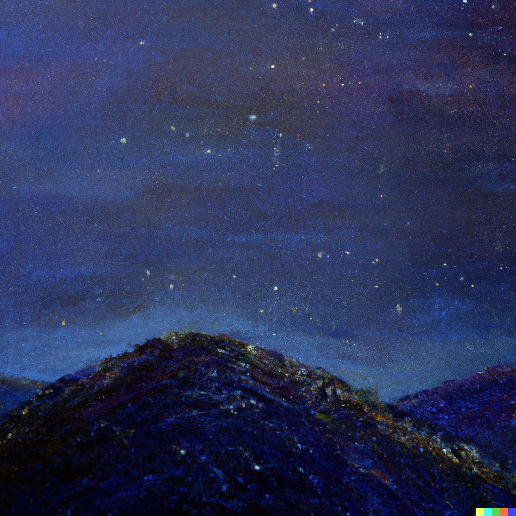Figure 5. original prompt: oil painting of a hill facing toward the stars. Middle image prompt: a girl sitting on a grassy hill looking at the stars. Right image prompt: A bunch of people including (insert character) staring at the stars on a hill. Figures from .

Zoom-out Inpainting. Zoom-out is another use of inpainting techniques by adding some padding to match up the image. Figure 6 shows the model (Right) the original image, (Middle) a padding version, and (Right) the model re-create the scene with a zoom-in rich color image from the same painting.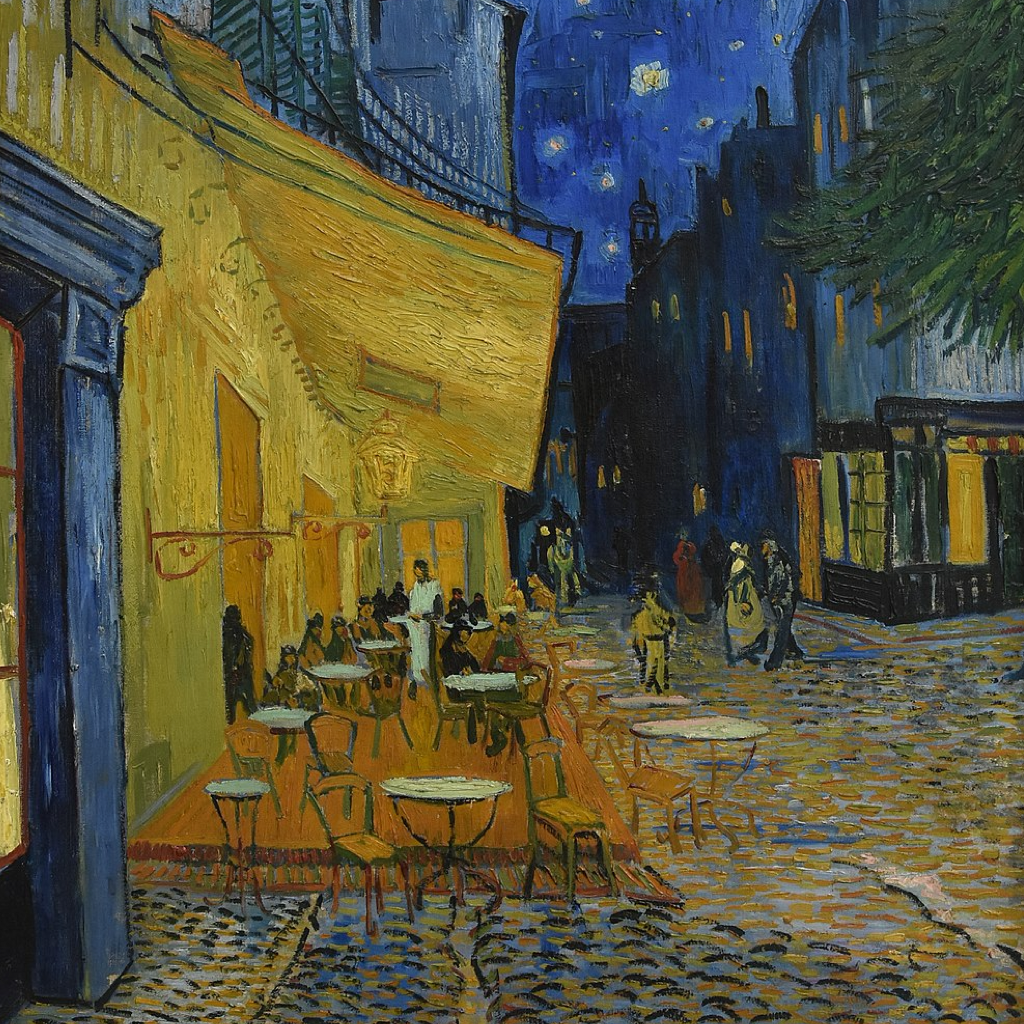Figure 6. (Left) original image. (Middle) zoom out with padding. (Right) prompt: Café Terrace at Night, Van Gogh. The model generates a fresh zoon-in vivid color image of the same painting. Figures from .

Research Application

A lot of applied research can take advantage of the high-level quality generated synthetic images. Next, we will discuss three types of research ideas that already exist but can take advantage of the generative model: (1) data generation, (2) caption evaluation, and (3) gender bias study.

Image Augmentation and Generation. Data generation can take advantage of the quality of these images that is similar to human pictures. Figure 7 and Figure 8 below show: (Left) image from COCO dataset and (Middle) and (Right) image from DALL-E 2 via synthetic prompt. In particular, we used Caption Transformer that is trained on a human-annotated COCO Caption dataset with beam search B= $$5$$ to generate different prompts. These images positive/negative can be used as training samples for supervised learning with labels (soft label), and also with unsupervised learning based contrastive learning objective function.

Caption Diversity Evaluation. Diversity based Caption evaluation is an important task in computer vision. Caption evaluation systems relies on traditional metrics like BLEU that disagrees with human judgment in most cases . In addition, diversity based metrics also are based $$n$$-gram counting , which cannot capture the semantic meaning of the caption. The evaluation can be done as follows: first, the model generates images using the image caption baseline beam search as a prompt. Secondly, an image matching via cosine similarity is used to compare the original image to the generated images. Figure 7 and Figure 8 show the original image from COCO (Left) and the most similar/dissimilar images generated by DALL·E $$2$$.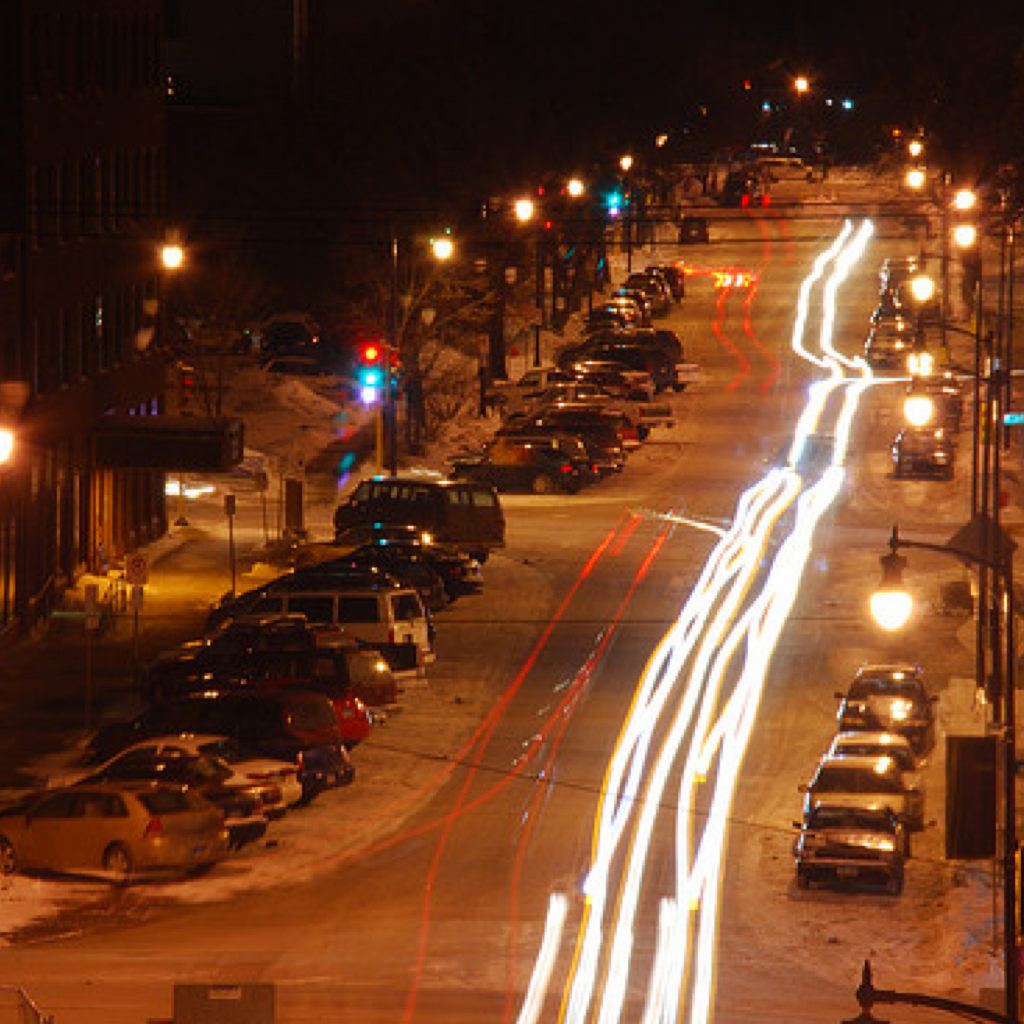Figure 7. (Left) The original image from COCO-caption . Middle image prompt: Caption Transformer best beam search k-5 a city street covered in traffic at night, Right image prompt: a city street filled with traffic and traffic lights.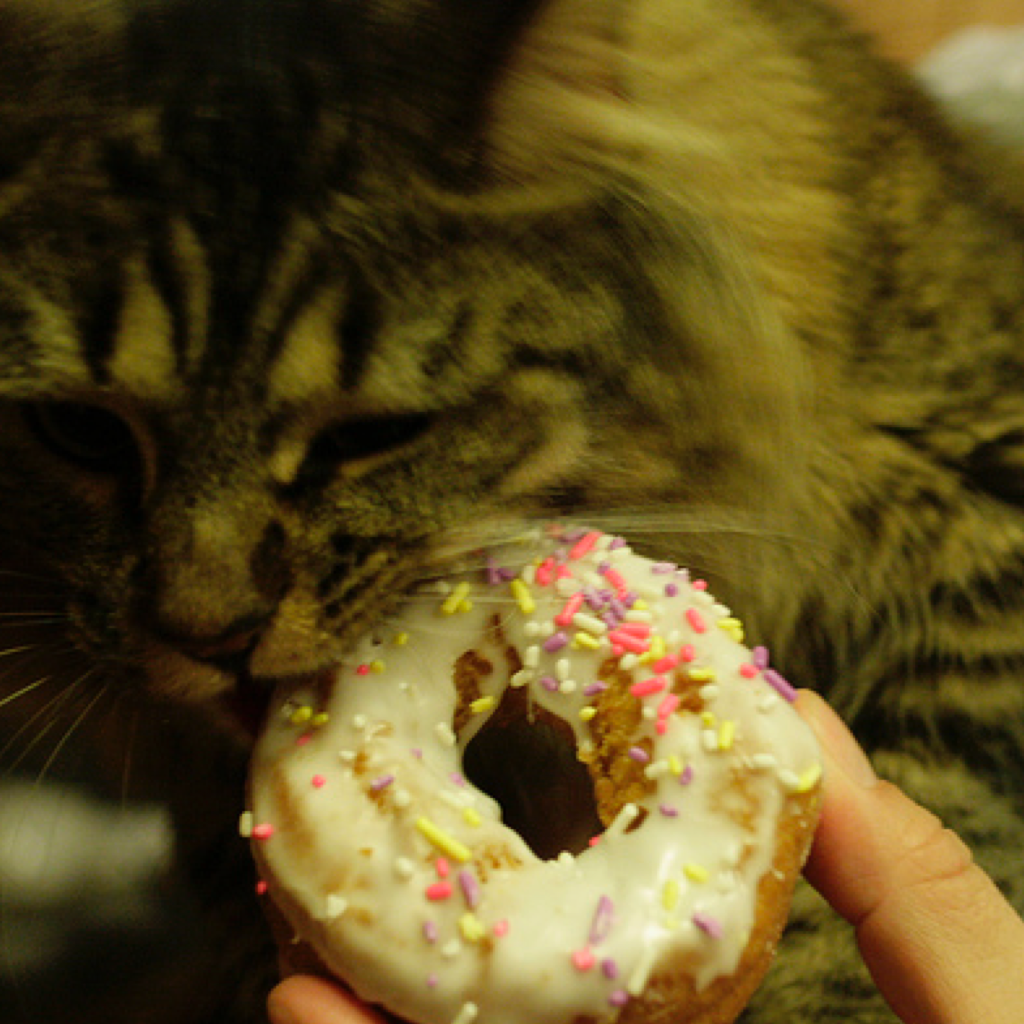Figure 8. (Left) The original image from COCO-caption . Middle image prompt: Caption Transformer best beam search k-5 a close up of a cat eating a doughnut, Right image prompt: a close up of a person holding a doughnut.

Gender Bias Evaluation. Another open research application is gender bias evaluation. Gender bias evaluation research in images is mostly active in image captioning task . The result shows that in image captioning, there is an existing gender bias ratio toward men 1:3 in COCO dataset . However, in this case, DALL·E 2 is trained using CLIP with a language model objective, therefore, the gender bias is amplified from both modalities vision and language. In Figure 9 and Figure 10, show that programmer and doctor professions have higher ratio towards man. Also, in most cases when there is a strong gender bias towards men, the women's images are enforced by a strong filter by the model, and therefore, the images look unnatural as shown in the Figures below, which indicates that openAI tried to balanced the gender bias. However, there is more research needs to be done, for example, the study of the direct and indirect bias (leakage bias) with a specific gender (e.g., food or kitchen associated with a woman picture).Figure 9. prompt: a doctor is treating a patient in a hospital. The bias is balanced and enforced, and thus the image of a woman doctor and patient look unnatural compared to a man doctor picture.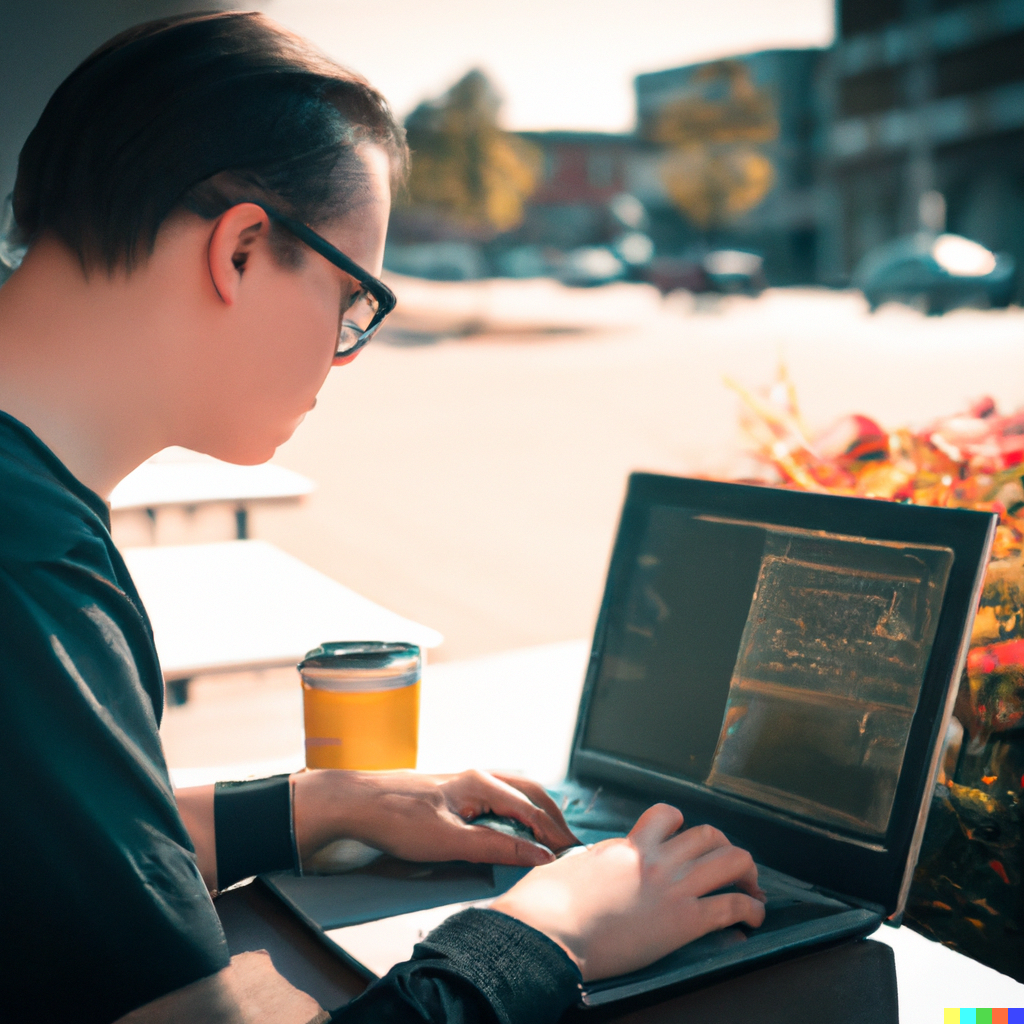Figure 10. (Left and Middle images) prompt: a programmer is writing some code outdoor and drinking some coffee. (Right image) prompt: a programmer is writing some code in Starbucks and drinking coffee. The bias is balanced and enforced by a filter to generate women's images, and thus the image looks unnatural.

## Summary#

In this short blog, we discuss DALL·E 2 a text-conditioned image generation model. The model can generate semantically plausible photorealistic images given a text prompt. The image generation relies on the semantic information from the text, such as a related object, background, etc. Also, we review some of its built-in inpainting features, such as image editing and zoom-out. In addition, we show some research directions that the model can benefit from.